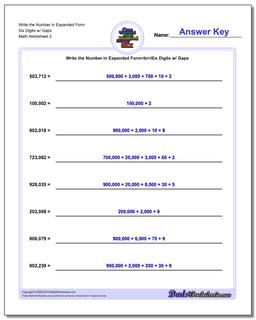# Math Worksheets: Standard, Expanded and Word Form: Standard, Expanded and Word Form: Write the Number in Expanded Form Six Digits w/ Gaps (Third Worksheet)## Write the Number in Expanded Form Six Digits w/ Gaps (Third Worksheet)

PropertyValue
DescriptionWrite the Number in Expanded Form Six Digits w/ Gaps: These are expanded form worksheets for converting numbers from standard numeric notation into conventional expanded form where the place value multiplier and the digit are combined. (Third Worksheet)
Resource TypeWorksheet